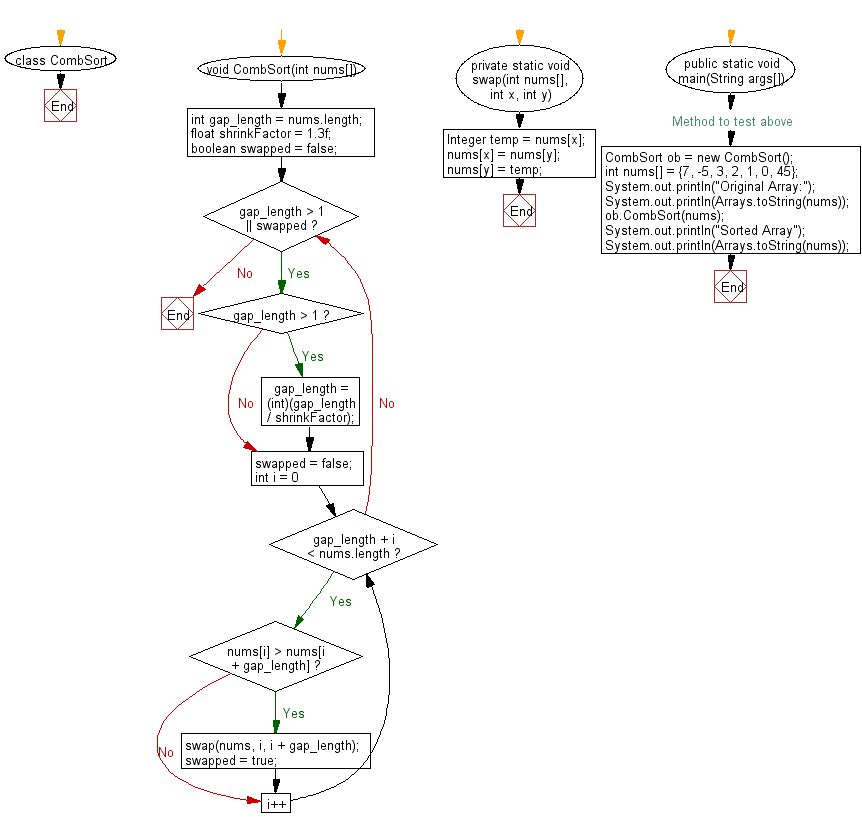﻿ Java exercises: CombSort Algorithm - w3resource# Java Exercises: CombSort Algorithm

## Java Sorting Algorithm: Exercise-11 with Solution

Write a Java program to sort an array of given integers using the CombSort Algorithm.

The Comb Sort is a variant of the Bubble Sort. Like the Shell sort, the Comb Sort increases the gap used in comparisons and exchanges. Some implementations use the insertion sort once the gap is less than a certain amount. The basic idea is to eliminate turtles, or small values near the end of the list, since in a bubble sort these slow the sorting down tremendously. Rabbits, large values around the beginning of the list do not pose a problem in bubble sort.
In bubble sort, when any two elements are compared, they always have a gap of 1. The basic idea of comb sort is that the gap can be much more than 1.

Visualization of comb sort :Animation credits : Jerejesse

Sample Solution:

Java Code:

``````import java.util.Arrays;
class CombSort {
void CombSort(int nums[]){
int gap_length = nums.length;
float shrinkFactor = 1.3f;
boolean swapped = false;

while (gap_length > 1 || swapped) {
if (gap_length > 1) {
gap_length = (int)(gap_length / shrinkFactor);
}

swapped = false;

for (int i = 0; gap_length + i < nums.length; i++) {
if (nums[i] > nums[i + gap_length]) {
swap(nums, i, i + gap_length);
swapped = true;
}
}
}
}
private static void swap(int nums[], int x, int y) {
Integer temp = nums[x];
nums[x] = nums[y];
nums[y] = temp;
}
// Method to test above
public static void main(String args[])
{
CombSort ob = new CombSort();
int nums[] = {7, -5, 3, 2, 1, 0, 45};
System.out.println("Original Array:");
System.out.println(Arrays.toString(nums));
ob.CombSort(nums);
System.out.println("Sorted Array");
System.out.println(Arrays.toString(nums));
}
}

```
```

Sample Output:

```Original Array:
[7, -5, 3, 2, 1, 0, 45]
Sorted Array
[-5, 0, 1, 2, 3, 7, 45]
```

Flowchart:Java Code Editor:

What is the difficulty level of this exercise?

﻿

## Java: Tips of the Day

Array vs ArrayLists:

The main difference between these two is that an Array is of fixed size so once you have created an Array you cannot change it but the ArrayList is not of fixed size. You can create instances of ArrayLists without specifying its size. So if you create such instances of an ArrayList without specifying its size Java will create an instance of an ArrayList of default size.

Once an ArrayList is full it re-sizes itself. In fact, an ArrayList is internally supported by an array. So when an ArrayList is resized it will slow down its performance a bit as the contents of the old Array must be copied to a new Array.

At the same time, it's compulsory to specify the size of an Array directly or indirectly while creating it. And also Arrays can store both primitives and objects while ArrayLists only can store objects.

Ref: https://bit.ly/3o8L2KH